Journal of Vibroengineering

Published: 30 June 2019

# Discussion on stability analysis and support technology of surrounding rock of gob-side entry retaining

Wenxiang Zheng1
Huiqiang Duan2
1, 2Institute of Mining and Coal, Inner Mongolia University of Science and Technology, Baotou, 014010, Inner Mongolia
Corresponding Author:
Wenxiang Zheng
Views 62

#### Abstract

In order to further analyze the stability and control mechanism of surrounding rock in the gob-side entry retaining, the slip instability coefficient ${k}_{1}$ and compression failure coefficient ${k}_{2}$ are proposed by the mechanical analysis of the established filling body mechanics model, and when both ${k}_{1}$ and ${k}_{2}$ are less than 1, the roadside filling body can keep stable. The mechanical model of main roof is analyzed by the static equilibrium method, and the formula for calculating the maximum roof subsidence is deduced. Based on the data of haulage gateway of No. 21201 coal face of Juncheng Coal Mine, using the formula to calculate the amount of roof subsidence at the junction point of filling body and the roof, and proposes two methods to reduce roadway floor heave by increasing the roadside filling width and transferring the surrounding rock stress to the gob. Finally, the corresponding surrounding rock control technology is proposed according to the surrounding rock deformation characteristics during roadway excavation, roadway retention and roadway reuse, and then carries out field monitoring and evaluation. The results show that after adopting the corresponding supporting technology, the surrounding rock of the gob-side entry retaining has been effectively controlled from the excavation to the reuse period with the coal face advancing, the roof-to-floor convergence of roadway solid coal side is less than 220 mm, the roof-to-floor convergence of filling body side is less than 280 mm, and the convergence on the both sides of the roadway is about 190 mm. At the same time, the calculated roof subsidence with the junction point of filling body and the roof is similar to the field monitoring results, which achieves the expected control effect, and the research contents provides a certain reference for the support technology of the gob-side entry retaining.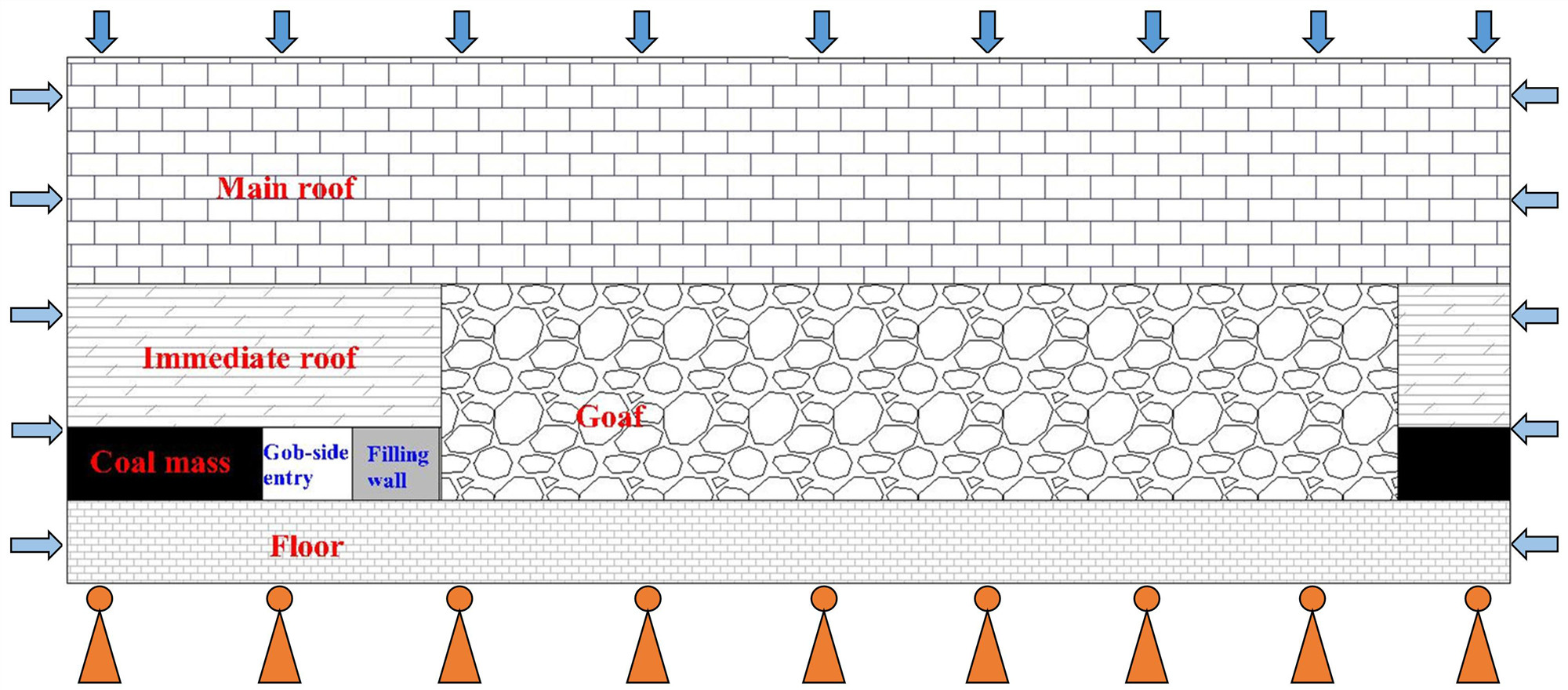#### Highlights

• By establishing the mechanical model of filling body, the slip instability coefficient k1 and the compressional failure coefficient k2 are proposed.
• By establishing the main roof mechanical model of gob-side entry retaining, the roadway roof subsidence is analyzed, then the formula for calculating the roadway roof subsidence is derived.
• To propose the surrounding rock control technology of gob-side entry in three stages of roadway excavation, retention and reuse.

## 2. Stability analysis of gob-side entry retaining surrounding rock

### 2.1. Establishment of the surrounding rock structure model of the gob-side entry retaining

With the advancement of the coal face and the gob area being filled with caving gangue, the roof of the gob-side entry retaining is swung and subsidence with the elastic-plastic boundary of the body of solid roadside as the rotating shaft. After reaching the tensile limit strength of the roof, the roof of the roadway is pulled down, forming large and small structures jointly carried by the solid roadside and the gob gangue. The large structure of the gob-side entry retaining refers to the relatively stable diagonal span beam structure formed by the caving of the main roof and above rock; the small structure refers to the single cantilever beam structure formed by the immediate roof. The stability of small structure is the premise and basis for the stability of large structure. The surrounding rock structure model of gob-side entry retaining is established as shown in Fig. 1.

### 2.2. Stability analysis of roadside filling body

In this paper, only the roadside filling body is taken as the research object, and the following is defined: the direction perpendicular to the roadway trend and parallel to the direction of the roadway floor is defined as the $X$ direction, the vertical direction is defined as the $Y$ direction, and the roadway trend is defined as $Z$ direction. The mechanics theory is used for analysis [17-20]. If the rotation angle of roadway roof is $\theta$, and the vertical stress that acting on the upper boundary of filling is $\sigma$, then the stress can be decomposed into ${\sigma }_{x}$ and ${\sigma }_{y}$, and, ${\sigma }_{x}=\sigma \mathrm{s}\mathrm{i}\mathrm{n}\theta$, ${\sigma }_{y}=\sigma \mathrm{c}\mathrm{o}\mathrm{s}\theta$, while the stress that acting on the floor of the filling body is ${\sigma }_{y}=\sigma \mathrm{c}\mathrm{o}\mathrm{s}\theta$. According to the characteristics of roadside filling body construction and the law of roof movement, it can be considered that the displacement in the $Z$ direction is $w=$ 0, which is the plane strain problem. The mechanical model of the roadside filling body is shown in Fig. 2.

Fig. 1The surrounding rock structure model of the gob-side entry retaining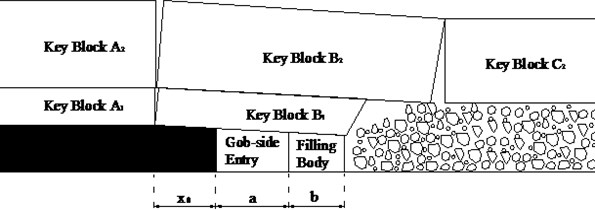Fig. 2The mechanical model of roadside filling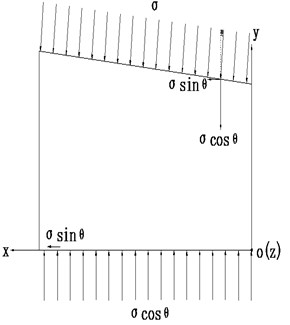As can be seen from Fig. 2 that the stress components ${\sigma }_{x}$ has two functions, one is to cause transverse deformation of the filling body; the other is to cause the filling body to slip along the floor hard platform to the roadway as a whole, which is also the main function of the stress component. There are two main functions of the stress component ${\sigma }_{y}$, one is to make the filling body produce vertical compression deformation, and the filling body is transverse deformed under the Poisson effect; the second function is to limit the slip caused by the shear stress ${\sigma }_{x}$ by the floor hard platform.

(1) Slip instability determination.

In order to avoid the slippage of the roadside filling body along the floor hard platform of the filling area, the request is $\mu \sigma \mathrm{c}\mathrm{o}\mathrm{s}\theta >\sigma \mathrm{s}\mathrm{i}\mathrm{n}\theta$, and define the following formula:

1
${K}_{1}=\frac{\sigma \mathrm{s}\mathrm{i}\mathrm{n}\theta }{\mu \sigma \mathrm{c}\mathrm{o}\mathrm{s}\theta }=\frac{\mathrm{s}\mathrm{i}\mathrm{n}\theta }{\mu \mathrm{c}\mathrm{o}\mathrm{s}\theta },$

where: ${K}_{1}$ is the slip instability coefficient; $\sigma$ is the vertical stress acting on the surface of filling body; $\theta$ is the roof rotation angle; $\mathrm{\mu }$is the friction coefficient between the filling body and the roadway floor.

When ${K}_{1}<$ 1, the roadside filling body will not slip; when ${K}_{1}=$ 1, the roadside filling body is in a critical stable state; when ${K}_{1}>$ 1, the roadside filling body will produce slip instability.

It can be known that $\mu$ under the constant condition, the rotation angle $\theta$ of the roof is the key factor that affecting whether the filling body sliding or not. With increasing of the angle $\theta$, the shear sliding force between the filling body and the floor hard platform increases, while the stability of filling body decreases. The control of the rotation angle $\theta$ can be controlled by increasing the filling body width of the roadway and increasing the stiffness of the filling body.

(2) Compression failure determination.

The size of $\sigma$ is closely related to the strata number of forming large structures and the length of cantilever beam. When the strata number of forming large structures remains unchanged, and when the filling width is constant, the bigger the length of cantilever beam, and the $\sigma$ is greater; when the length of cantilever beam is constant, the filling width is large, then $\sigma$ is small. Therefore, in order to avoid failure to the roadside filling body, it is required to: ${\sigma }_{t}>\sigma \mathrm{c}\mathrm{o}\mathrm{s}\theta$, and define the following formula:

2
${K}_{2}=\frac{\sigma \mathrm{c}\mathrm{o}\mathrm{s}\theta }{{\sigma }_{t}}.$

where: ${K}_{2}$ is the compression failure coefficient; ${\sigma }_{t}$ is the compressive strength of the filling body.

When ${K}_{2}<$ 1, the roadside filling body will not cause failure; when ${K}_{2}=$ 1, the roadside filling body is in a critical failure state; when ${K}_{2}>$ 1, the roadside filling body will produce compression failure. Therefore, in order to prevent the failure of filling body, it can be controlled by increasing the filling body width of the roadway and increasing the stiffness of the filling body.

According to the comprehensive analysis, in order to ensure the stability of the roadside filling body, both ${K}_{1}$ and ${K}_{2}$ should be less than 1, and in order to prevent the failure of filling body, it can be controlled by increasing the filling body width of the roadway and increasing the stiffness of the filling body.

## 3. Deformation analysis and control of roof and floor of gob-side entry retaining

### 3.1. Analysis of roof subsidence of the gob-side entry retaining

Since the main roof of gob-side entry retaining acts on the coal and rock mass below in a given deformation manner, when the solid roadside, the roadside filling body, and the gob gangue can balance the load of overlying strata, the roof of gob-side entry will no longer subsidence, and the establishment that the mechanical model of main roof in the gob-side entry retaining is shown in Fig. 3.

Fig. 3The mechanical model of main roof in the gob-side entry retaining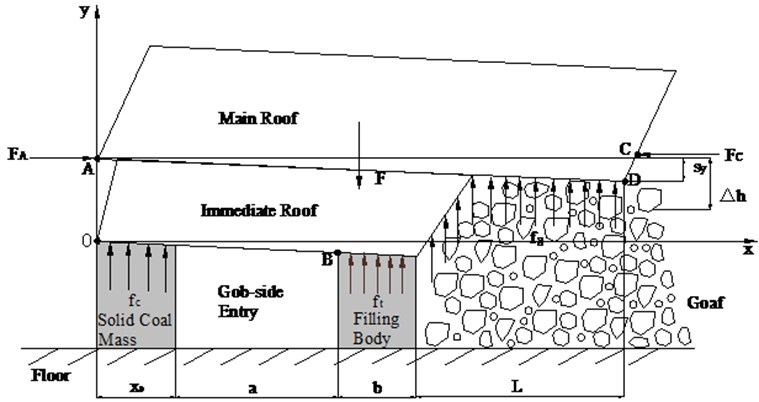Assuming that the main roof is pulled off at the point A of elastic-plastic boundary in the solid roadside and takes it as the rotation axis to gyrate and subsidence [21-24], after immediate roof caving in the gob, and the vertical displacement of contact with gangue at point D of main roof is:

3
${s}_{y}=m-\left[m\left(1-\eta \right){K}_{m}+{H}_{z}\left({K}_{z}-1\right)\right].$

At point B, the subsidence of gob-side entry retaining is:

4
$\mathrm{\Delta }{h}_{B}={s}_{y}×\frac{{x}_{0}+a}{{x}_{0}+a+b+L},$

where, $m$ is the mining height, m; $\eta$ is the mining rate of coal face; ${K}_{m}$ is the crushing expansion coefficient of coal body, which is taken as 1.15-1.25; ${H}_{z}$ is the caving roof thickness, m; ${K}_{z}$ is the crushing expansion coefficient of caving roof, and it is taken as 1.15-1.25; ${x}_{0}$ is the plastic zone range of solid roadway coal body, m; $a$ is the width of gob-side entry retaining, m; $b$ is the width of roadside filling body, m; $L$ is the length of main roof cantilever beam, m.

From Eq. (4), it can be seen that the mining height and the thickness of main roof are the key factors to affect the subsidence movement of main roof. Under the condition that the thickness of main roof is constant, when the mining height is small, the movement space of main roof is small, and the roadside support resistance is also small. In the case of mining height is constant, if the thickness of main roof is large and the roof can fully fall, the movement space of main roof is small, and the roadside support resistance is also small.

Since the gob gangue has a certain compressibility, the actual subsidence of main roof $\mathrm{\Delta }h$ is larger than the value expressed by the Eq. (4). It is assumed that the horizontal force ${F}_{A}$ acting at the main roof point A is equal to the horizontal force ${F}_{C}$ at the main roof point C, while the direction is opposite. There is no horizontal displacement of main roof fractured and no moment influence, but only there is the vertical displacement. It is analyzed by the limit equilibrium method, and when the support resistance provided by the solid roadside support, the roadside support and the gob caving gangue can balance the weight of overlying broken rock strata, the main roof no longer moves [3, 25-27], at this time there are:

5
$F={\sum }_{i=o}^{n}{\mathrm{\gamma }}_{i}{h}_{i}{L}_{i}={\int }_{0}^{{x}_{0}}{f}_{c}dx+{\int }_{{x}_{0}+a}^{{x}_{0}+a+b}{f}_{t}dx+{\int }_{{x}_{0}+a+b}^{{x}_{0}+a+b+L}{f}_{g}dx,$

where: $F$ is the gravity of the unit width of overlying fractured rock strata, N; $n$ is the number of caving strata; ${f}_{c}$ is the support resistance of per unit width of solid roadside along the roadway direction, Pa; ${f}_{t}$ is the support resistance of per unit width of roadside filling body along the roadway direction, Pa; ${f}_{g}$ is the support resistance of per unit width of caving gangue in the gob along the roadway direction.

For the convenience of calculation, it is assumed that the equivalent elastic modulus of the solid roadside, the filling body, and the gangue is ${E}_{c}$, ${E}_{t}$, ${E}_{g}$ [28-30] then:

6
${\int }_{0}^{{x}_{0}}{f}_{c}dx=\frac{{E}_{c}{x}_{0}^{2}/2}{H\left({x}_{0}+a+b+L\right)}={E}_{c}{\epsilon }_{c}{x}_{0},$
7
${\int }_{0+a}^{{x}_{0}+a+b}{f}_{t}dx=\frac{{E}_{t}b\left({x}_{0}+a+b/2\right)}{H\left({x}_{0}+a+b+L\right)}\mathrm{\Delta }h={E}_{t}{\epsilon }_{t}b,$
8
${\int }_{{x}_{0}+a+b}^{{x}_{0}+a+b+L}{f}_{g}dx=\frac{{E}_{g}L\left({x}_{0}+a+b+L/2\right)\left(\mathrm{\Delta }h-{s}_{y}\right)}{\left[m\left(1-\eta \right){K}_{m}+{H}_{z}\left({K}_{z}-1\right)\right]\left({x}_{0}+a+b+L\right)}={E}_{g}{\epsilon }_{g}L,$

where: ${E}_{c}{\epsilon }_{c}$ can be expressed by the residual compressive strength ${\sigma }_{c}^{*}$ of coal body; ${E}_{t}{\epsilon }_{t}$ and ${E}_{g}{\epsilon }_{g}$ can be represented by ${\sigma }_{t}^{*}$ and ${\sigma }_{g}^{*}$ respectively, and the specific values can be determined by laboratory test. By substituting Eqs. (6), (7) and (8) into Eq. (5), the static equilibrium calculation formula for the final roof subsidence position of gob-side entry retaining is obtained as follows:

9
$F=\sum _{i-0}^{n}{\gamma }_{i}{h}_{i}{L}_{i}={\sigma }_{c}^{\mathrm{*}}{x}_{0}+{\sigma }_{t}^{\mathrm{*}}b+{\sigma }_{g}^{\mathrm{*}}L.$

Assuming no subsidence and slip at point A, the gob gangue can be completely compacted, and the number of floor heave of gob gangue is 0, it can be considered that the maximum subsidence of the roof at point D should be equal to the height of coal face minus the thickness of floating coal, that is:

10
${s}_{yzd}=m\left(1-\eta \right).$

At this time, the amount of roof subsidence of gob-side entry retaining at point B is:

11
$\mathrm{\Delta }{h}_{Bzd}={s}_{yzd}×\frac{{x}_{0}+a}{{x}_{0}+a+b+L}=\frac{m\left(1-\eta \right)\left({x}_{0}+a\right)}{{x}_{0}+a+b+L}.$

It can be seen from the Eq. (11) that when mining height and the width of the retaining roadway are constant, the longer the cantilever beam of main roof is, the smaller the roof subsidence will be. But with the increasing of cantilever beam length of main roof, the load acting on the filling body also increases, and at the same time, the stress transmitted to the surrounding rock of roadway floor increases accordingly, if the stress acting on the floor exceeds its compressive strength, the floor surrounding rock will cause damage, causing the floor heave of roadway.

The following data were obtained from the field surrounding rock of haulage gateway of No. 21201 coal face of Juncheng Coal Mine and the laboratory: $m=$ 3.3 m; 0.78; ${x}_{0}=$ 2.1 m; $a=$ 3.8 mm; $b=$ 1.5 m; $L=$ 8.9 m. We can obtain the amount of roof subsidence at the point B of filling body by the Eq. (11) about is 138 mm.

According to the characteristics of floor heave deformation, the following two methods for controlling the floor heave are proposed:

(1) Increase the roadside filling width.

The increase of roadside filling width is beneficial to the decrease of stress in the surrounding rock of roadway floor; however, from the economic point of view, the roadside filling width should be as small as possible, therefore, the compromise plan is that the economically reasonable roadside filling width should not exceed 80 % of the cost of roadway drivage, and does not affect the normal advancement of coal face.

(2) Transfer the surrounding rock stress to the gob.

Due to the fact that the gob caving gangue has the characteristics of higher compression bearing capacity, it is advantageous to control the roadway floor heave by allowing certain deformation of the coal and rock mass in the solid roadside and the filling body beside the roadway to transfer the stress of overlying strata to the gob. This kind of roadside support method requires the yield to be the main stage in the early stage and the pressure resistance as a supplement, in the latter period, the opposite is true. Although this method will increase the amount of roof subsidence and the plastic zone of solid roadside in the early stage, but it fully utilizes the bearing capacity of the gob caving gangue, which is beneficial to reduce the roadway floor heave.

## 4. The surrounding rock control technology of gob-side entry retaining

The gob-side entry retaining needs to go through three stages, namely: roadway excavation, mining of the first coal face and mining of the second coal face, the corresponding supporting technology should also fully consider the deformation characteristics of surrounding rock in these three stages.

### 4.1. Surrounding rock control during roadway excavation

During the roadway excavation, the surrounding rock deformation of roadway is relatively small, the plastic zone is small, and the required support resistance is also small. However, after the gob-side entry retaining, due to the strong dynamic pressure, the plastic zone of surrounding rock is expanding rapidly, and the separation between roof strata is easy to occur. At this time, the control effect of reinforcement support on surrounding rock becomes very limited, therefore, the basic support design in the roadway should be based on meeting the support requirements during the retaining roadway period.

As an active support method, bolt support can effectively improve the peak strength and residual strength of anchor solids and increase the bearing capacity of surrounding rock. At the same time, the bolt itself has a large elongation rate (not less than 15 %), which allows and can adapt to large deformation of surrounding rock while maintaining high resistance.Especially, the elongation of the left-turning bolt can reach 25 %, and the corresponding bearing capacity is increased by more than 10 % compared with other bolts of the same specification. Therefore, the left-turning bolt should be used as the basic support form in the gob-side entry retaining, and the anchor cable should also be reinforced. In addition, reasonable pre-stress should be applied to the anchor cable and the bolt to realize the synchronous bearing of both, so as to maximize the supporting capacity of the whole support system.

### 4.2. Surrounding rock control during retaining roadway

Roadside support is the key support technology for surrounding rock control of gob-side entry retaining, the roadside support must ensure the integrity of immediate roof above the filling body and the strata separation from the main roof, and at the same time, the cutting roof can be realized in the gob, it should also have an appropriate amount of shrinkage to accommodate the roof subsidence and rotation. The roadside support is divided into two parts: the roof support and the roadside filling support.

1) Filling area roof support. The main function of the roof support in the filling area is to improve the integrity and damage resistance of immediate roof, so that avoiding the separation strata between the immediate roof and the main roof, and achieve the coordinated deformation of the same strata in the roadway, so its support form should be consistent with the basic support form in the roadway, and the left-turning bolt with mesh belt should be adopted.

2) Roadside filling support. As the support body on the side along the gob-side entry retaining, it is the most important influencing factor to ensure the shape of the final roadway section, and also is the key factor for the formation of stable large and small structure of the gob-side entry retaining.

For the gob-side entry retaining under the condition of hard roof, the flexible supporting roadside filling method is adopted. Through the early yield action, the roof will rotation and subsidence with the coal body at the elastic-plastic boundary of solid coal mass, and the internal stress field is easily formed, and the formation of internal stress field avoids the influence of the high-level roof to the gob-side entry retaining. At this time, the support object is only the large and small structure of the surrounding rock within the range of internal stress field, which is beneficial to maintenance.

For the gob-side entry retaining with soft roof, the roof cantilever beam length is short. In order to ensure that the roof is not cut off in the roadway, the roadside filling body must have higher bearing capacity in the initial stage of filling, and its stiffness can meet the requirement of synchronous deformation with the roadside for coal and rock mass. Therefore, under the condition of soft roof, a greater rigidity roadside filling method such as concrete pouring should be adopted.

(2) Strengthening support in the roadway.

Due to the continuous movement of surrounding rock of gob-side entry retaining, it is difficult to ensure that the roadway roof is not separated strata and fall by relying solely on the basic support in the roadway. Therefore, the role of strengthening support in the roadway is to prevent the separated strata and fall of roof, and ensure the stability of roadway roof, single hydraulic prop with articulated top beam are preferred for strengthening support in roadways.

### 4.3. Surrounding rock control during roadway reuse

After one mining impact, the surface of gob-side entry retaining has become soft and broken. When the mining is carried out at the next coal face, the deformation of surrounding rock near the peak of speed-up support pressure is extremely large, and almost lost self-supporting ability. Therefore, the maintenance at this time to ensure that the roadway does not roof fall and can meet the most basic ventilation and pedestrian conditions required for the production of coal face, without excessive emphasis on the overall control of the deformation of the surrounding rock. The support measure is to increase the strength and density of the speed-up support pressure for the mining roadway within the affected area. The support form can be selected as single hydraulic prop with articulated top beam or the $\mathrm{\Pi }$ type steel beam.

## 5. Field monitoring and evaluation

In order to reasonably evaluate the supporting reliability of the gob-side entry retaining, a measuring station is set at every intervals of 5 m in the roadway retaining section to observe the deformation of roof-floor and both sides of roadway. Fig. 4 shows that the variation of surrounding rock deformation with the advancing of working face after roadside filling of gob-side entry retaining.

Fig. 4The surrounding rock deformation with the advancing of working face after roadside filling of gob-side entry retaining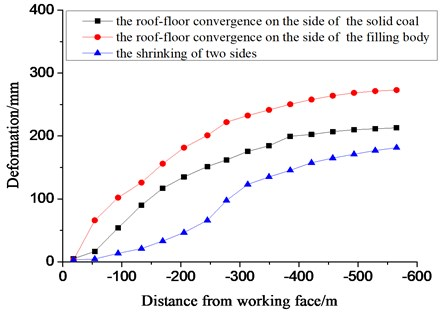It can be seen from Fig. 4 that the roof and floor approaching rate of gob-side retaining roadway decreases gradually with the advancing of working face. When the coal face is advanced over 350 m, the deformation rate is significantly reduced and enters a slow creep state. In the initial stage of gob-side entry retaining, the both sides were basically free of deformation. After the coal face was advanced over 50 m, the deformation rate of both sides increased, while after the coal face was advanced over 350 m, the deformation rate of both sides began to decrease again. The deformation of both sides showed the characteristics of smooth, accelerated and creep. When the measured station is 560 m away from the coal face, the roof-floor convergence on one side of the solid coal roadway is less than 220 mm, the roof-floor convergence on one side of the filling body is less than 280 mm, and the shrinking of two sides is about 190 mm. The shape of roadway section is relatively regular, which can meet the requirements of next coal face mining. At the same time, the amount of roof subsidence at the junction of filling body and the field monitoring is close to the calculated result.

## 6. Conclusions

1) By establishing the mechanical model of filling body, the slip instability coefficient ${k}_{1}$ and the compressional failure coefficient ${k}_{2}$ are proposed, when both ${k}_{1}$ and ${k}_{2}$ are less than 1, the roadside filling body can be kept stable, to prevent the failure of filling body, it can be controlled by increasing the width of roadway filling body and increasing the strength of filling body.

2) By establishing the main roof mechanical model of gob-side entry retaining, the roadway roof subsidence is analyzed, then the formula for calculating the roadway roof subsidence is derived, and an example calculation is carried out.

3) Proposed the method of increasing the filling width of roadway and transferring the surrounding rock stress to the gob to reduce the roadway floor heave, and the control technology of gob-side entry retaining in three stages of roadway excavation, roadway retention and roadway reuse was proposed.

4) Through field monitoring, from the initial stage to the reuse after the gob-side entry retaining, the roof-floor convergence on one side of the solid coal roadway is less than 220 mm, the roof-floor convergence on one side of the filling body is less than 280 mm, and the both sides convergence is about 190 mm, and the amount of roof subsidence at the junction of filling body and the field monitoring is close to the calculated result.

#### Acknowledgements

This project was supported by a High School Science research project of the Inner Mongolia Autonomous Region (NJZY16158, NJZY18151, NJZY17175), Inner Mongolia University of Science and Technology and Innovation Fund Project (2015QDL01), the National Natural Science Foundation of China (51774131).

#### Cited by

Determination of Reasonable Width of Filling Body for Gob-Side Entry Retaining in Mining Face with Large Cutting Height
Research on Deformation and Failure Control Technology of a Gob-Side Roadway in Close Extra-Thick Coal Seams